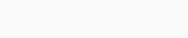# Predict food delivery time using machine learning in Python

This tutorial will help you to learn food delivery time prediction using machine learning in Python.

At present in the world, many businesses are going online and people give orders online instead of personally going and buy. In this online order system especially food product is very popular and increase their use very well. There are many applications available for online orders of foods that supply the food at home, for example, Zomato, Swiggy, etc. These applications get the order online as per your selection of foods and selection of restaurants and then their appointed delivery person picks up that food and delivers at the mention location. The time of delivery is an important factor and therefore we understand the model to predicts estimated time for the delivery of food.

## Building a model for predicting food deliver time

Here, we implement a model in the four following steps.

Step-1 Importing required libraries

```import pandas as pd
import numpy as np
import matplotlib.pyplot as plt
import seaborn as sns
from sklearn.ensemble import RandomForestClassifier
from sklearn.preprocessing import LabelEncoder
from sklearn.metrics import accuracy_score
from sklearn.model_selection import train_test_split```

Step-2 Reading and transforming the data

```train_data=pd.read_excel('Data_Train.xlsx')
`train_data.head()`

Output:Transforming data into useful information.

```train_data['Restaurant']=LabelEncoder().fit_transform(train_data['Restaurant'])
train_data['Location']=LabelEncoder().fit_transform(train_data['Location'])
train_data['Cuisines']=LabelEncoder().fit_transform(train_data['Cuisines'])
train_data['Average_Cost']=pd.to_numeric(train_data['Average_Cost'].str.replace('[^0-9]',''))
train_data['Minimum_Order']=pd.to_numeric(train_data['Minimum_Order'].str.replace('[^0-9]',''))
train_data['Rating']=pd.to_numeric(train_data['Rating'].apply(lambda x: np.nan if x in ['Temporarily Closed','Opening Soon','-','NEW'] else x))
train_data['Votes']=pd.to_numeric(train_data['Votes'].apply(lambda x: np.nan if x=='-' else x))
train_data['Reviews']=pd.to_numeric(train_data['Reviews'].apply(lambda x: np.nan if x=='-' else x))
train_data['Delivery_Time']=pd.to_numeric(train_data['Delivery_Time'].str.replace('[^0-9]',''))```

Checking for null values and replace them with useful information.

`sns.heatmap(train_data.isnull(),cmap='viridis')`

Output:```train_data['Rating']=train_data['Rating'].fillna(train_data['Rating'].median())
train_data['Reviews']=train_data['Reviews'].fillna(train_data['Reviews'].mean())
train_data['Average_Cost']=train_data['Average_Cost'].fillna(train_data['Average_Cost'].mean())
train_data.tail()```

Output:Step-3 Making model

Creating input and output. Split that into train and test data.

```X=train_data.drop('Delivery_Time',axis=1)
y=train_data['Delivery_Time']
X_train,X_test,y_train,y_test=train_test_split(X,y,test_size=0.3,random_state=51)
```

Initialize the model and fitting data into it. Here we use the Random forest classifier in our model.

```RFC=RandomForestClassifier(n_estimators=1000,criterion='entropy',random_state=51)
RFC.fit(X_train,y_train)```

Step-4 Predict and evaluating the model

```pred=RFC.predict(X_test)
```

Calculate the accuracy of the model.

`accuracy_score(y_test,pred)`

Output:## Dataset for Predicting food delivery time

The dataset is available at Kaggle. The data set contains three excel files.

• Data_Train.xlsx
• Data_Test.xlsx
• Sample_Submission.xlsx

You can download the dataset from here: Predicting Food Delivering Time

#### Conclusion

Use of Model with help of Python using Machine Learning for

• In-time delivery
• Predict food deliver time

### One response to “Predict food delivery time using machine learning in Python”

1. Chandrika Ajjada says:

Can I know how this will work in R? That will be of help.